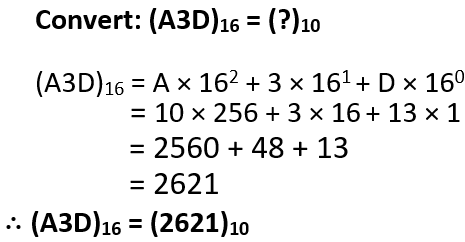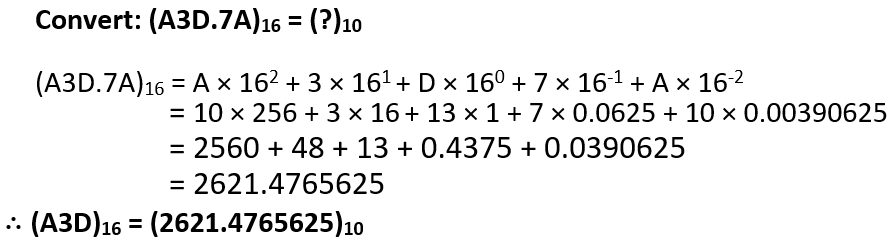# Hexadecimal to Decimal Conversion# Whole Number Conversion
﻿→ Hexadecimal Number is converted to decimal number by calculating the sum of the product of each hexadecimal digit(if there is any digit from A-F, then that should be replaced by corresponding numeric value) and its corresponding place value in term of power of 16 which begins from 0 and increases for integer part.
Example;Fractional Conversion(Only for Grade-XI)
→ The integer part for the fractional Hexadecimal Number is converted by the same method shown above; for fractional part we calculate the sum of the product of each hexadecimal digit and its corresponding place value in term of power of 16 from -1 which decreases as we move right to fractional part.
Example;